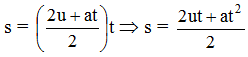Ex﻿

# 1. Motion

#### Equation of Motion

Motion under uniform acceleration
(a) 1st Equation of Motion
Consider a body having initial velocity 'u'. Suppose it is subjected to a uniform acceleration 'a' so that after time 't' its final velocity becomes 'v'. Now we know,or v = u + at …..(i)

(b) 2nd Equation of Motion
Suppose a body has an initial velocity 'u' and uniform acceleration 'a' for time 't' so that its final velocity becomes 'v'. The distance travelled by moving body in time 't' is 's' then the average velocity = (v + u)/2.
Distance travelled = Average velocity × time
s =s = ut +at2 … … (ii)
(c) 3rd Equation of Motion
Distance travelled = Average velocity x time
s =……(iii)
from equation (i), we haveSubstituting the value of t in equation (iii), we get2as = v2 – u2
or
v2 = u2 + 2as … . (iv)
The equations of motion under gravity can be obtained by replacing acceleration by acceleration due to gravity (g) and can be written as follows :
When the body is coming towards the centre of earth
(a) v = u + gt
(b) h = ut +gt2
(c) v2 = u2 + 2gh

When a body is thrown upwards with some initial velocity, then a retardation produced due to attraction of the earth. In equations of motion, a is replaced by (–g) and thus equations become.
(a) v = u – gt
(b) h = ut –gt2
(c) v2 = u2 – 2gh

If you want to give information about online courses to other students, then share it with more and more on Facebook, Twitter, Google Plus. The more the shares will be, the more students will benefit. The share buttons are given below for your convenience.
×

#### NTSE Physics (Class X)

• Electricity
• Magnetic Effect of Curent
• Light Reflection
• Light Refraction
• Human Eye & Colourful World
• Source of Energy
• Motion

#### NTSE Physics (Class IX)

• Force & laws of motion
• Work and Energy
• Gravitation
• Sound

SHOW CHAPTERS